# NR HDL MIB Recovery

This example shows how to design a 5G NR synchronization signal block (SSB) decoding and master information block (MIB) recovery model optimized for HDL code generation and hardware implementation.

### Introduction

The Simulink® models described in this example are fixed-point HDL optimized implementations of SSB decoding and MIB recovery for 5G NR frequency range 1 (FR1). This example is one of a related set which show the workflow for designing and deploying a 5G NR cell search and MIB recovery algorithm to hardware. The complete workflow is shown.Each step in this workflow is demonstrated by one or more related examples.

1. The MATLAB Golden Reference Algorithm step consists of the NR Cell Search and MIB and SIB1 Recovery (5G Toolbox) example, which shows the floating-point golden reference algorithm.

2. The MATLAB Hardware Reference Algorithm step consists of the NR HDL Downlink Receiver MATLAB Reference example, which models hardware friendly algorithms and generates test waveforms. This MATLAB® code operates on vectors and matrices of floating-point data samples and does not support HDL code generation.

3. The Simulink Fixed-Point Implementation Model step consists of multiple examples. The NR HDL Cell Search example demonstrates a 5G cell search Simulink subsystem that uses the same algorithm as the MATLAB reference. The NR HDL MIB Recovery example (this example) adds a broadcast channel decoding and MIB recovery subsystem. The NR HDL SIB1 Recovery example adds a subsystem that recovers the SIB1 resource grid, and shows how to decode its output using MATLAB reference code. The NR HDL MIB Recovery for FR2 example shows cell search and MIB recovery models which have been extended to support FR2. These models operate on fixed-point data and are optimized for HDL code generation.

4. The Simulink SoC Deployment Model step consists of the Deploy NR HDL Reference Applications on SoCs examples, which build on the fixed-point implementation models and use hardware support packages to deploy the algorithms on hardware.

For a general description of how MATLAB and Simulink can be used together to develop deployable models, see Wireless Communications Design for FPGAs and ASICs.

MIB recovery requires SSB detection, demodulation, and decoding. This example focuses on SSB decoding. SSB detection and demodulation are described in the NR HDL Cell Search example. This example introduces the SSB decoding Simulink model and uses the MATLAB reference to generate test input and verify the behavior of the model. Then, the example describes a Simulink model that combines SSB detection, demodulation, and decoding to recover MIB from a baseband waveform.

After an SSB has been detected and demodulated, it needs to be decoded to extract the MIB contents. SSB decoding requires demodulation reference signal (DMRS) search, channel estimation and phase equalization, and broadcast channel (BCH) decoding steps as shown in the figure below.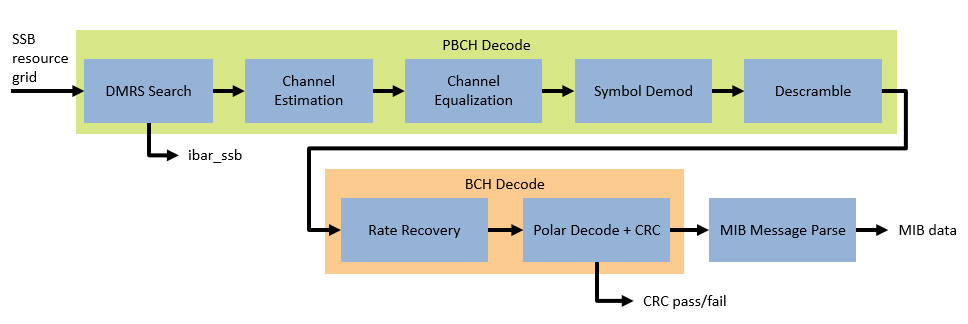### File Structure

This example uses these files.

• `nrhdlSSBDecoding.slx`: This Simulink model uses the `nrhdlSSBDecodingCore` model reference to simulate the behaviour of the SSB decoding part of the MIB recovery process.

• `nrhdlMIBRecovery.slx`: This Simulink model combines the processing of the SSB detector and the SSB decoder into an integrated model illustrating the complete MIB recovery process. This model uses the `nrhdlSSBDetectionFR1Core` and `nrhdlSSBDecodingCore` model references.

• `nrhdlSSBDecodingCore.slx`: This model reference implements the SSB decoding algorithm.

• `nrhdlSSBDetectionFR1Core.slx`: This model reference implements the SSB detection algorithm.

• `nrhdlReceiverData.sldd`: This Simulink data dictionary contains bus objects that define the buses contained in the example models.

MATLAB code

• `runSSBDecodingModel.m`: This script uses the MATLAB reference to implement the cell search algorithm, then runs the `nrhdlSSBDecoding` Simulink model. The script verifies the operation of the model using 5G toolbox and the MATLAB reference code.

• `runMIBRecoveryModel.m`: This script uses the MATLAB reference to perform the search mode of the SSB detection algorithm, then runs the `nrhdlMIBRecovery` Simulink model. The script verifies the operation of the model using 5G toolbox and the MATLAB reference code.

• `nrhdlexamples`: Package containing the MATLAB reference code and utility functions for verifying the implementation models.

### NR HDL SSB Decoding

This figure shows the `nrhdlSSBDecoding` model. The top level of the model reads the signals from the MATLAB base workspace, passes them to the SSB Decoding subsystem, and writes the outputs back to the workspace. The ParseMIB subsystem takes the pbchPayload and interprets the bit fields to produce the MIB parameter outputs.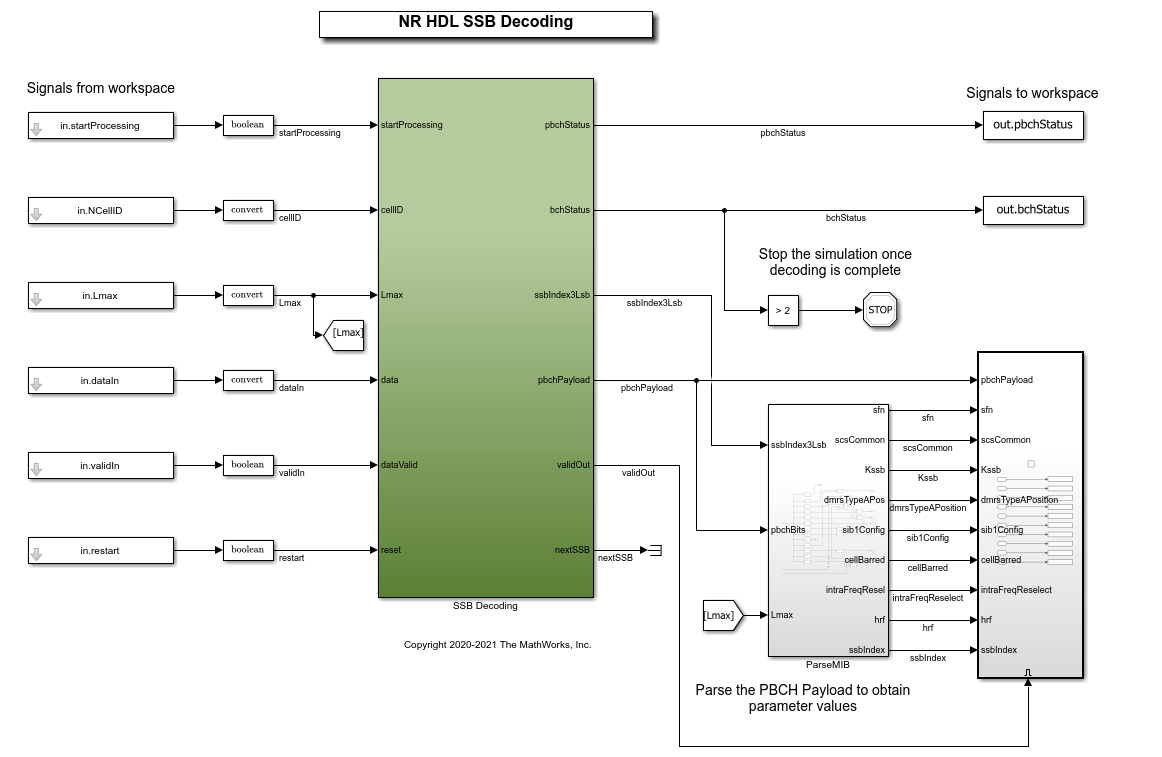### SSB Decoding Interface

The SSB Decoding subsystem contains an instance of the `nrhdlSSBDecodingCore` model reference. This section describes the inputs and outputs of that model.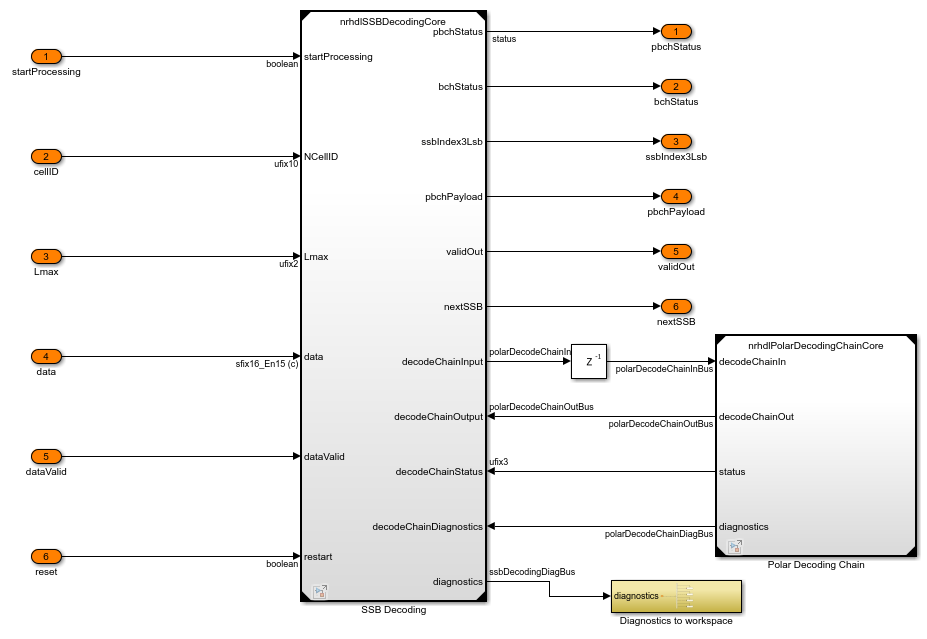Inputs

• startProcessing: 1-bit control signal which indicates when all data has been written and that cellID and Lmax are valid.

• NCellID: 10-bit unsigned number which provides cell ID number for the detected SSB.

• Lmax: 2-bit unsigned number which indicates the maximum number of SSBs in a burst. A value of 0 indicates 4 SSBs and a value of 1 indicates 8 SSBs.

• data: 16-bit signed complex-valued signal carrying the 4 OFDM symbols of the SSB.

• dataValid: 1-bit control signal to validate data.

• reset: 1-bit control signal to reset the processing.

Outputs

• pbchStatus: 2-bit unsigned value indicating the progress of the PBCH decoding operation. See below for more information on the possible values of this signal.

• bchStatus: 3-bit unsigned value indicating the progress of the BCH decoding operation. See below for more information on the possible values of this signal.

• ssbIndex3Lsb: 3-bit unsigned value that is the 3 least significant bits of the SSB index calculated by the DMRS search process and Lmax.

• pbchPayload: 32-bit unsigned value that contains the MIB and additional PBCH timing data.

• validOut: 1-bit control signal to validate ssbIndex3Lsb and pbchPayload.

• nextSSB: 1-bit control signal to indicate when the core can begin processing the next SSB. Can be used to pace inputs for back-to-back SSB decodes.

• diagnostics: Bus containing diagnostic signals.

PBCH Status Signal States

• `0`: idle

• `1`: reading in data for SSB grid

• `2`: performing DMRS search

• `3`: performing PBCH symbol demodulation

BCH Status Signal States

• `0`: idle

• `1`: performing rate recovery

• `2`: performing polar decoding

• `3`: CRC error (end state)

• `4`: CRC pass, MIB detected (end state)

### SSB Decoding Model Reference Structure

This diagram shows the top level of the `nrhdlSSBDecodingCore` model. The input data is 4 OFDM symbols for the synchronization signal block (SSB), with the values scaled within the range +/-1. The model starts processing when all of the SSB data has been input to the model and startProcessing is asserted. The startProcessing signal also indicates that the NCellID and Lmax inputs are valid.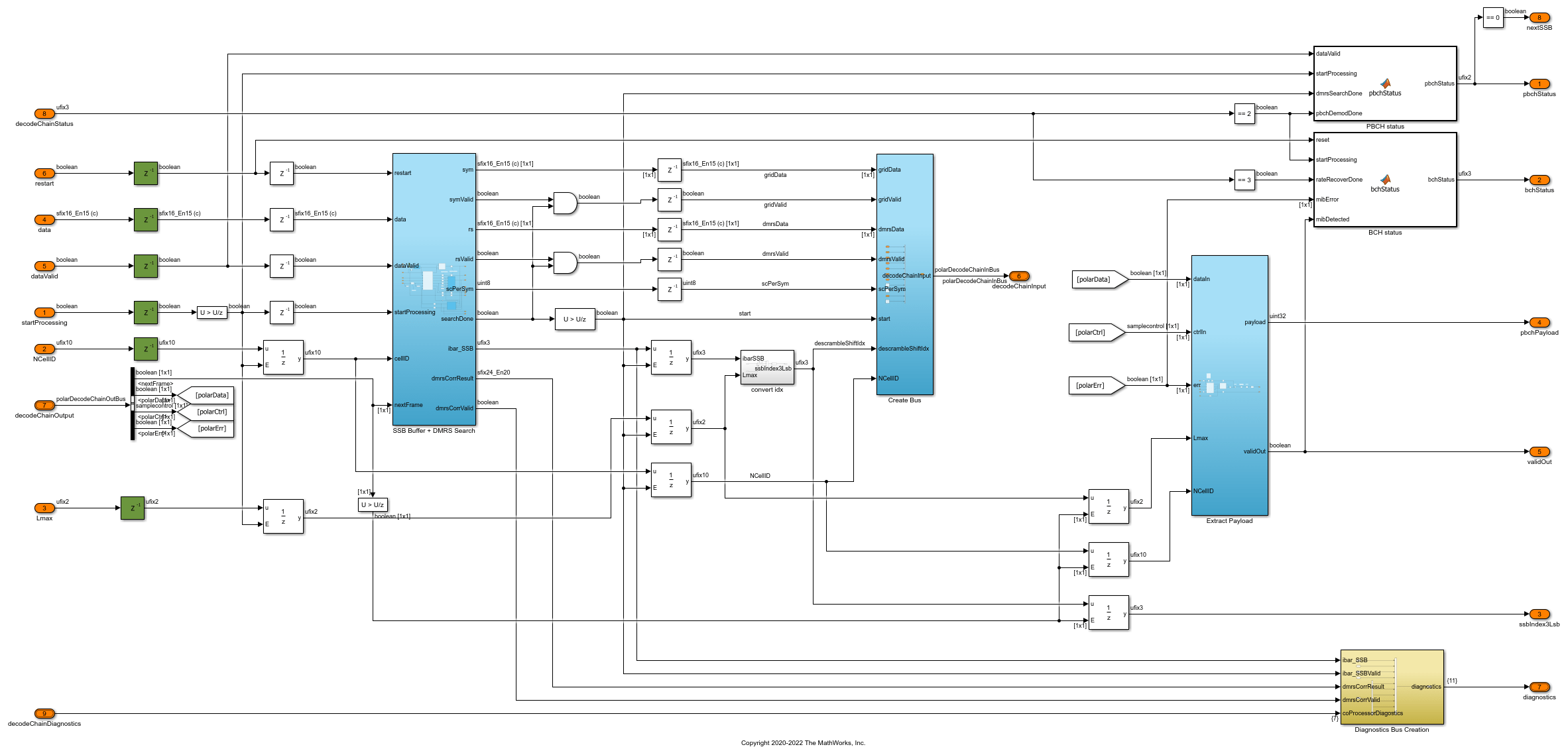The PBCH processing subsystem performs DMRS search, channel estimation and equalization, QPSK symbol demodulation, and descrambling. The output from the PBCH processing subsystem is passed to the BCH processing subsystem which performs rate recovery, polar decoding, and CRC decoding. The Diagnostics Bus Creation subsystem creates the diagnostics bus by concatenating the diagnostics from the PBCH and BCH processing subsystems.

### PBCH Processing Subsystem

The PBCH processing subsystem performs DMRS search, channel estimation and equalization, and QPSK demodulation and descrambling. Incoming data is stored in a RAM buffer where it is held until startProcessing is asserted, indicating that all required information is available to start the DMRS search process. The DMRS search reads the DMRS symbols from the RAM and correlates with the 8 possible DMRS sequences, selecting the strongest correlation value to determine ibar_SSB. Once the DMRS search has been completed ibar_SSB is used to generate the reference DMRS required for channel estimation. The reference DMRS is passed to the channel est + eq subsystem along with the received PBCH symbols and associated DMRS.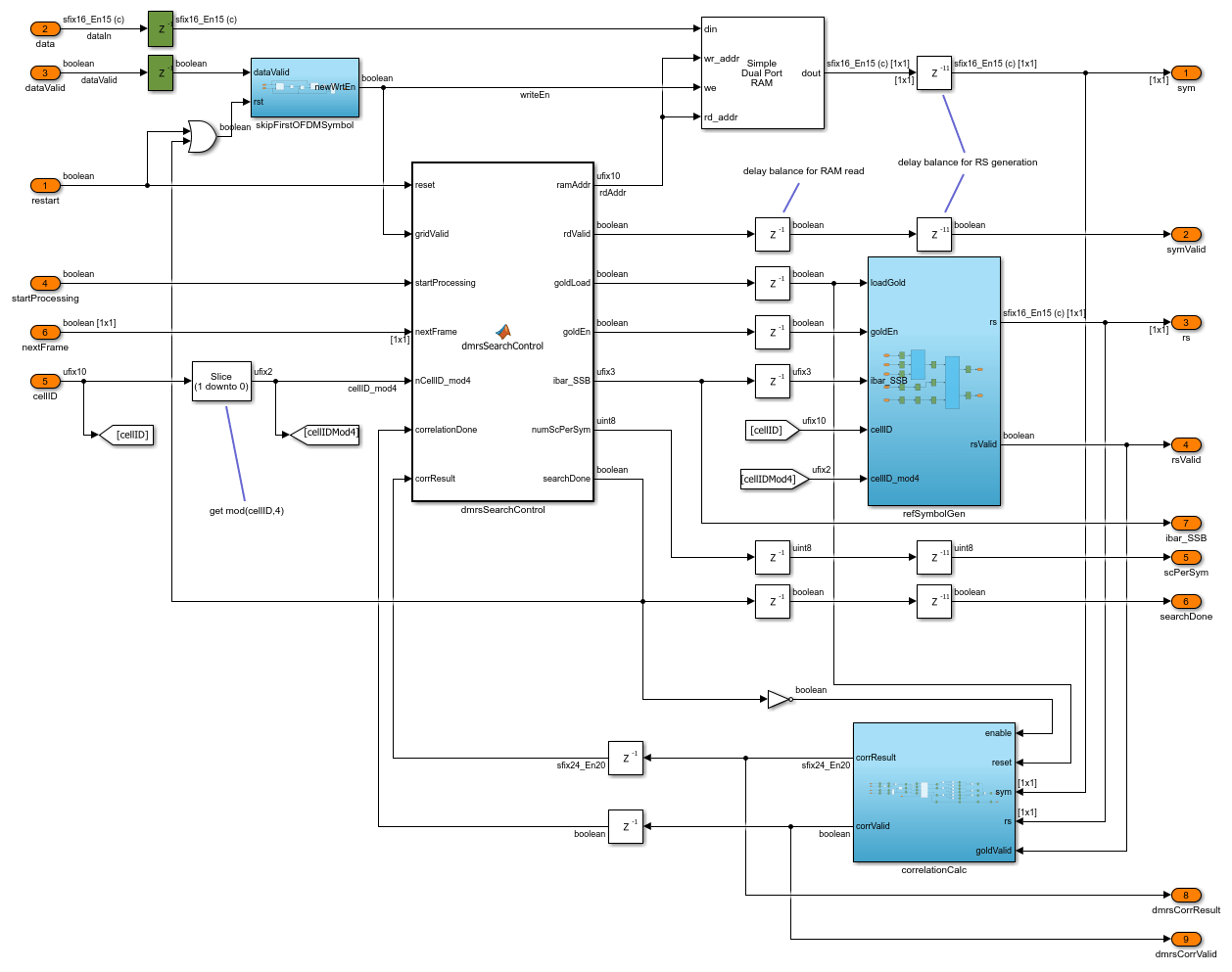The channel est + eq subsystem performs channel estimation using the received data and the reference DMRS. The channel estimate applies linear interpolation between DMRS locations within an OFDM symbol, but does not average across time in case of any residual carrier frequency offset. Phase equalization of the PBCH symbols is then performed, followed by QPSK demodulation and descrambling, using ibar_SSB and Lmax to calculate the descrambling sequence.

### BCH Processing Subsystem

The BCH processing performs rate recovery, polar decoding, and CRC decoding of the BCH. The rate recovery subsystem includes signal scaling and wordlength reduction to prepare the data for polar decoding. The scaled, rate-recovered soft bits are then passed to the NR Polar Decoder block, which also performs CRC decoding. The err output port from the NR Polar Decoder block indicates if decoding was successful or encountered any errors. The extract payload subsystem performs descrambling and deinterleaving of the payload bits.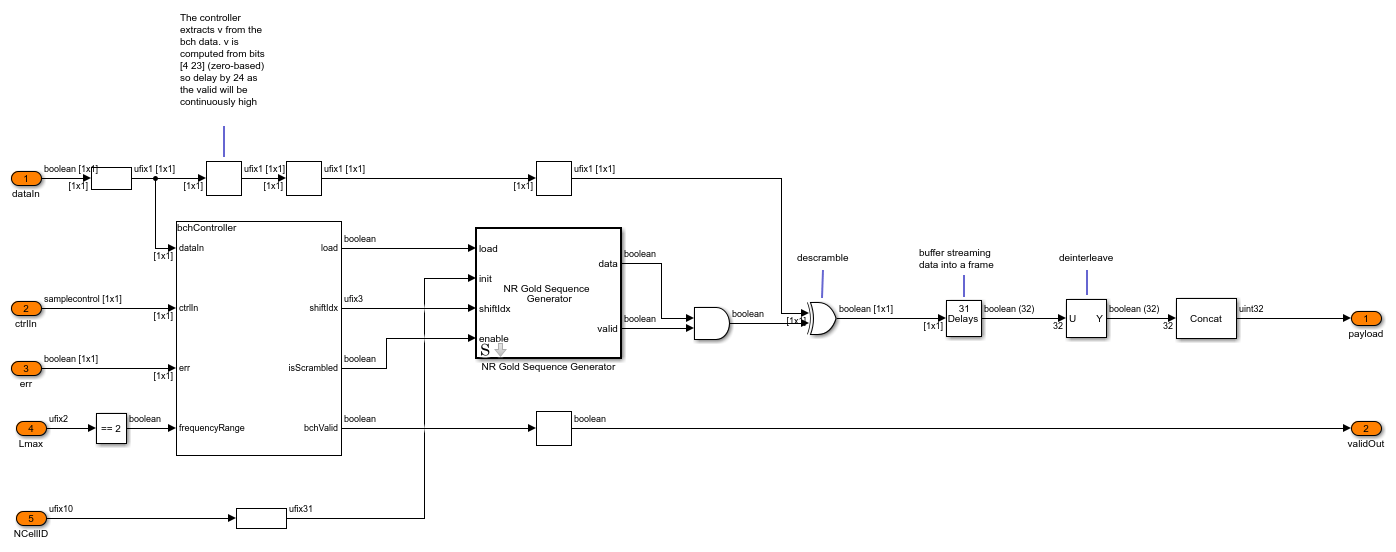### SSB Decoding Simulation Setup

The block diagram shows the simulation setup implemented by this example. 5G Toolbox™ functions are used to generate a test waveform. MATLAB reference code for the SSB detector is then used to search for and demodulate the strongest SSB within the waveform. This result provides test input for the SSB decoding stage. The test data is passed to both MATLAB and Simulink implementations, and the outputs are compared to verify the operation of the Simulink model.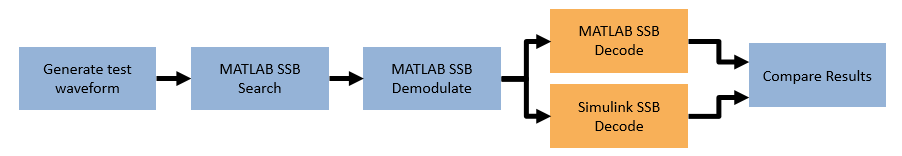### SSB Decoding Simulation

Use the `runSSBDecodingModel` script to run an SSB decoding simulation. The script displays its progress at the MATLAB command prompt. The final results of decoding the SSB in MATLAB and Simulink are displayed, showing that they match exactly. Plots of the DMRS search correlation strength and the equalized PBCH QPSK symbols show that the signals from MATLAB and Simulink match closely.

`runSSBDecodingModel;`
```Generating test waveform. Selected Simulation case: Simulation Case SSB Pattern Subcarrier Spacing Common PDCCH Config SIB1 SNR dB Strongest SSB index Lmax _______________ ___________ _________________________ _________________ ______ ___________________ ____ "SimCase 1" "Case C" 30 164 50 4 8 Searching for SSBs using the MATLAB reference. Demodulating the strongest SSB using the MATLAB reference. Decoding the SSB using the MATLAB reference. MIB successfully decoded by MATLAB reference Decoding the SSB using the Simulink model. Running nrhdlSSBDecoding.slx ### Starting serial model reference simulation build ### Model reference simulation target for nrhdlSSBDecodingCore is up to date. Build Summary 0 of 1 models built (1 models already up to date) Build duration: 0h 0m 2.3755s .......... MIB successfully decoded by Simulink model MATLAB decoded information pbchPayload: 17637376 ssbIndex: 3 hrf: 0 err: 0 mib: [1×1 struct] Simulink decoded information pbchPayload: 17637376 ssbIndex: 3 hrf: 0 err: 0 mib: [1×1 struct] MATLAB decoded MIB parameters NFrame: 0 SubcarrierSpacingCommon: 30 k_SSB: 0 DMRSTypeAPosition: 3 PDCCHConfigSIB1: 164 CellBarred: 0 IntraFreqReselection: 0 Simulink decoded MIB parameters NFrame: 0 SubcarrierSpacingCommon: 30 k_SSB: 0 DMRSTypeAPosition: 3 PDCCHConfigSIB1: 164 CellBarred: 0 IntraFreqReselection: 0 ```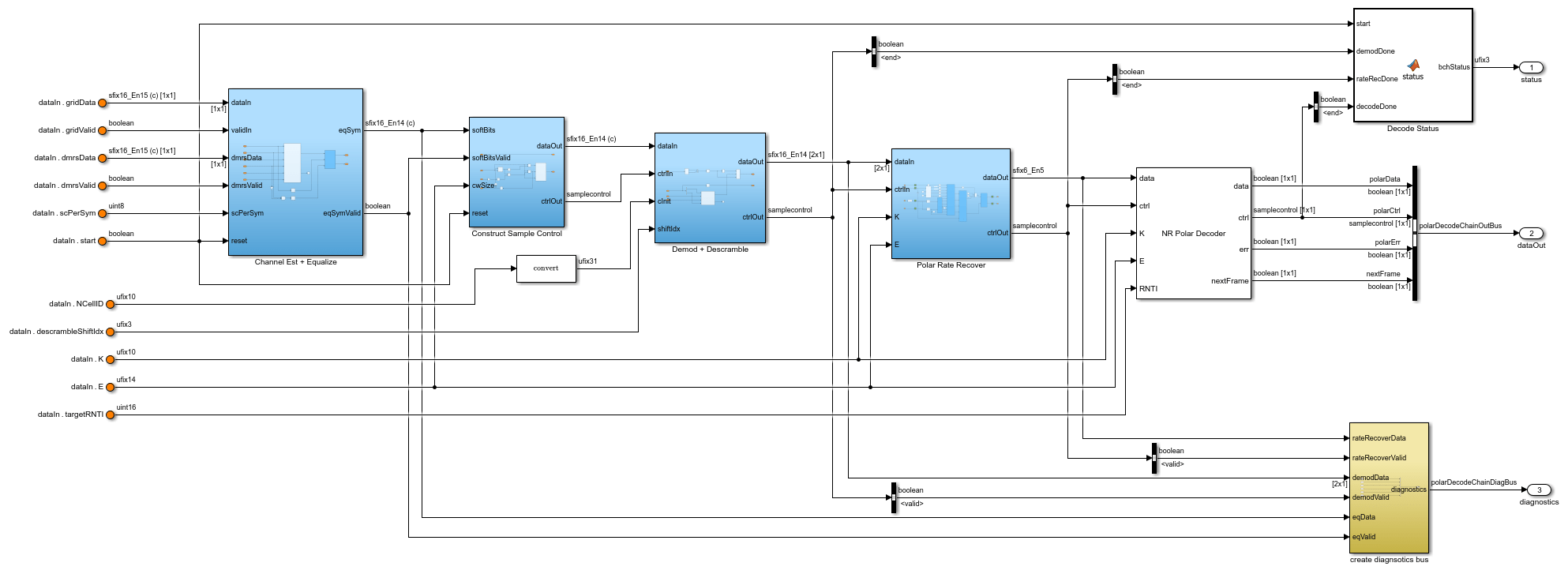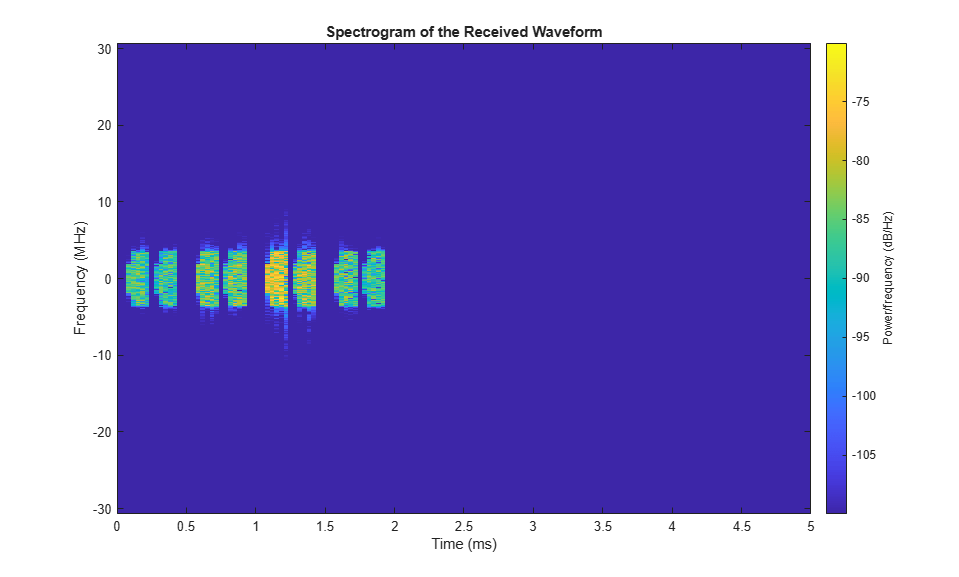### MIB Recovery Model

The `nrhdlMIBRecovery` model connects the two reference models for SSB Decoding and SSB detection (`nrhdlSSBDecodingCore` and `nrhdlSSBDetectionFR1Core`) to create a complete MIB recovery implementation. This model can be used to recover MIB from baseband 5G waveforms. The script `runMIBRecoveryModel` can be used to run this model and compare against the MATLAB reference. To reduce the processing time required the cell search part of the algorithm is performed in MATLAB then, once the strongest SSB has been determined, the Simulink model is used to re-acquire, demodulate, and decode the SSB.

The status signal from the detector is used to start the SSB decoder when it has reached state 8, indicating that demodulation is complete, SSS has been found, and the demodulated grid has been output. When the SSB decoder has the demodulated grid and received the startProcessing signal it will decode the SSB, outputting the PBCH payload which is then parsed to extract the MIB data.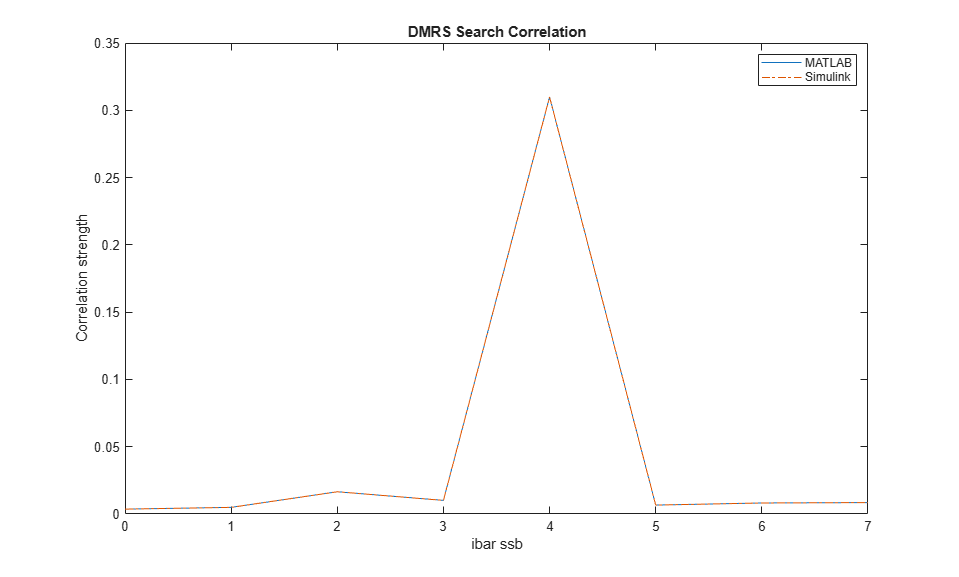### HDL Code Generation and Implementation Results

To generate the HDL code for this example, you must have an HDL Coder™ license. Use the `makehdl` and `makehdltb` commands to generate HDL code and an HDL test bench for `nrhdlSSBDecoding/SSB Decoding` or `nrhdlMIBRecovery/MIB Recovery` subsystems. The resulting HDL code was synthesized for a Xilinx® Zynq®-7000 ZC706 evaluation board. The table shows the post place and route resource utilization results. The design meets timing with a clock frequency of 150 MHz.

Resource utilization for nrhdlSSBDecoding model:

``` Resource Usage _______________ _____ Slice Registers 8297 Slice LUTs 11050 RAMB18 8 RAMB36 4 DSP48 37 ```

Resource utilization for nrhdlMIBRecovery model:

``` Resource Usage _______________ _____ Slice Registers 83231 Slice LUTs 40154 RAMB18 17 RAMB36 5 DSP48 245 ```